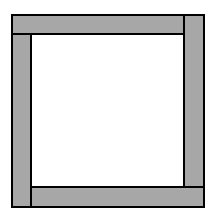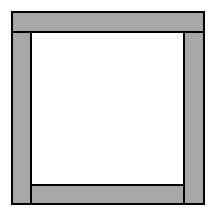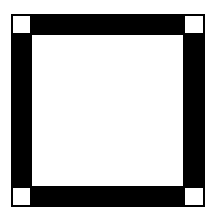### Home > CC1MN > Chapter 4 Unit 4 > Lesson cc14.1.2 > Problem4-17

4-17.

A team of students worked on problem 4-12. The team’s work is shown below. Unfortunately, the expressions, descriptions, and diagrams got mixed up! Match the counting method, word description, and diagram that describe the same strategy.

Counting
Methods

Word
Descriptions

Diagrams

1. $4·10−4$

1. Start in one corner and count 9 four times around the picture frame.

1.1. $10+9+9+8$

1. Take a side length of 10 four times and take away the four corners.

1.1. $9·4$

1. Take the entire $100$'s grid and subtract the inside part of the picture frame.

1.1. $(10·10)−(8·8)$

1. Take the top length, then add the two vertical sides and add the bottom.

1.$\text{c}\rightarrow 1 \rightarrow \text{A}$